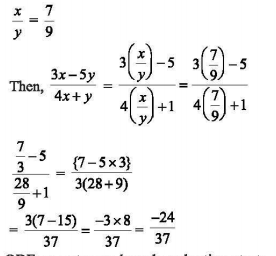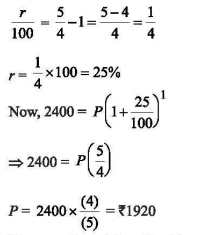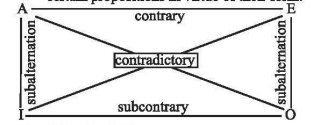# UGC NET Paper 1 December (Pack of 3) - UGC NET PAPER 1 3rd December 2019 Morning Shift

>>>>>>>>UGC NET PAPER 1 3rd December 2019 Morning Shift

• A

-24/37• B

-22/37• C

15/22• D

42/37• Option : A
• Explanation :• A

Semesterized examinations• B

Performance evaluation• C

Portfolio based evaluation• D

Learning outcome based evaluation• Option : D
• Explanation : OBE or outcome-based evaluation starts with clear definition of learning outcomes to be achieved followed by designing of curriculum and instruction on the same lines and assessment based on that to make sure the planned outcomes have been achieved or desired learning ultimately has happened. This is an innovative practice. The others mentioned are the traditional ones.

• A

Rs 1,900• B

Rs 1,910• C

Rs 1,915• D

Rs 1,920• Option : D
• Explanation :• A

Experimental research• B

Historical research• C

Survey research• D

Exegetic research• Option : A
• Explanation : The experimental method is a systematic and scientific approach to research in which the researcher manipulates one or more variables, and controls and measures any change in other variables. Hypothesis testing is a statistical analysis of experimental data for making statistical decisions about whether to accept or reject the null hypothesis. Every test in hypothesis testing produces the significance value for that particular test. If the significance value of the test is greater than the predetermined significance level, then the null hypothesis is accepted, if it is less, the null hypothesis is rejected.

• A

Contrary• B

Contradiction• C

Sub contrary• D

Subaltern• Option : C
• Explanation : The square of opposition shown below represents the logical relationships between certain propositions in virtue of their form.The following hold true:
I. Two propositions are contradictory when the truth of one implies the falsity of the other, and conversely.
2. Two propositions are contrary when they cannot both be true.
3. Two propositions are subcontrary when it is impossible for both to be false.
4. Two propositions stand in the relation of subalternation when the truth of the first (the superaltern) implies the truth of the second (the subaltern), but not conversely.